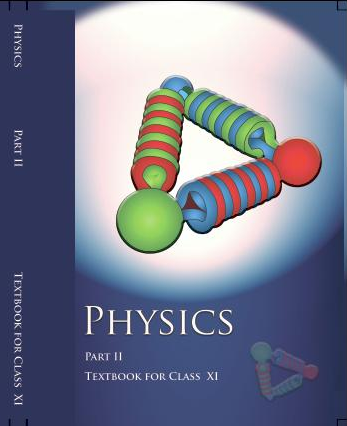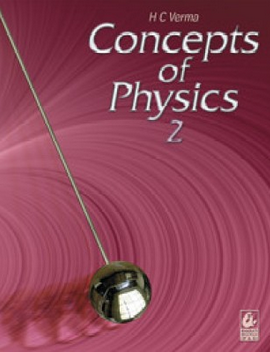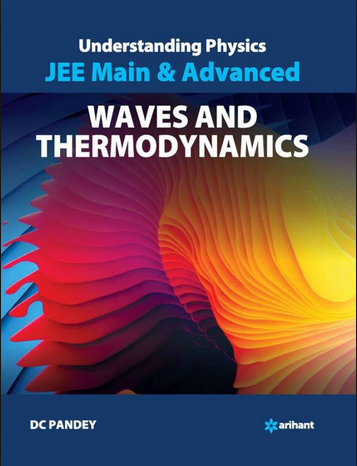# Behaviour of Perfect Gas and Kinetic theory (Weightage 1%)   Share

### Topics from Behaviour of Perfect Gas and Kinetic theory

• Equation of state of a perfect gas (13 concepts)
• Kinetic theory of gases : Assumptions, concept of pressure,kinematic interpretation of gases and rms speed of gas molecules (8 concepts)
• Concept of mean free path (3 concepts)
• Degrees of freedom (5 concepts)
• application to specific heat capacities of gases (2 concepts)
• Kinetic energy and temperature (1 concepts)
• Equation of state of a perfect gas, work done on compressing a gas (2 concepts)
• Kinetic theory of gases- assumptions, concept of pressure (13 concepts)
• Kinetic energy and temperature:rms speed of gas molecules: Degreesof freedom (9 concepts)
• Applications to specific heat capacities of gases: Mean free path, Avogadro'snumber (8 concepts)

### Important Books for Behaviour of Perfect Gas and Kinetic theory

•••Exams
Articles
Questions